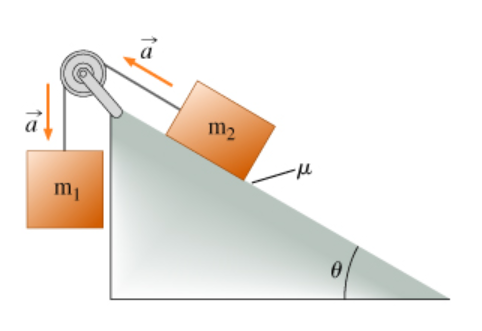# Problem: Block 1, of mass m1, is connected over an ideal (massless and frictionless) pulley to block 2, of mass m2, as shown. Assume that the blocks accelerate as shown with an acceleration of magnitude a and that the coefficient of kinetic friction between block 2 and the plane is θ. Find an expression for the ratio of the masses m1/m2.

###### FREE Expert Solution

Newton's second law:

$\overline{){\mathbf{\Sigma }}{\mathbf{F}}{\mathbf{=}}{\mathbf{m}}{\mathbf{a}}}$

For block 1:

m1g - T = m1a

m1 (g - a) = T

m1 = T/(g - a)

94% (376 ratings)###### Problem Details

Block 1, of mass m1, is connected over an ideal (massless and frictionless) pulley to block 2, of mass m2, as shown. Assume that the blocks accelerate as shown with an acceleration of magnitude a and that the coefficient of kinetic friction between block 2 and the plane is θ. Find an expression for the ratio of the masses m1/m2.